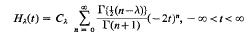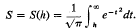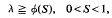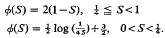Home

17.—An Upper Bound for the Largest Zero of Hermite's Function with Applications to Subharmonic Functions

Synopsis

Letbe Hermite's function of order λ and let h = h(λ) be the largest real zero of Hλ(t). SetIn this paper we establish the inequalitywhereEquality holds for S = ½. The result is also fairly accurate as S→0 and S→1. The proof is analytical except in the ranges −1·1 ≦ h ≦ −0·1 andwhere the argument is concluded by means of a computer.

The following deduction is made elsewhere [2, Theorem A]. If u(x) is subharmonic in Rm(m ≧ 2) and the set E where u(x) > 0 has at least k components, where k ≧ 2, then the order ρ of u(x) is at least ϕ(1/k). In particular, if ρ < 1, E is connected. This result fails for ρ = 1.

Hide All

Metrics

Full text viewsFull text views reflects the number of PDF downloads, PDFs sent to Google Drive, Dropbox and Kindle and HTML full text views.

Total number of HTML views: 0
Total number of PDF views: 0 *Loading metrics...

Abstract viewsAbstract views reflect the number of visits to the article landing page.

Total abstract views: 0 *Loading metrics...

* Views captured on Cambridge Core between <date>. This data will be updated every 24 hours.

Usage data cannot currently be displayed